# Electronics and Communication Engineering - Exam Questions Papers

31.

{ x(n) } is a real-valued periodic sequence with a period N. x(n) and X(k) form N-point. Discrete Fourier Transform (DFT) pairs. The DFT Y(k) of the sequence. y(n) =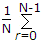x(r) X (n + r) is

 A. |X(k)|2 B.X(r) X(k + r) C. 1 D. 0

Explanation:

Direct property

Convolution in time domain ↔ Multiplication in frequency domain.

32.

The signal x(t) is described by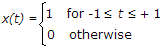Two of the angular frequencies at which its fourier transform becomes zero are

 A. p, 2p B. 0.5p, 1.5p C. 0, p D. 2p, 2.5p

Explanation:

X(jω) =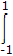1.e-jωt =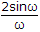At ω = 0 X(j0) = 2

At ω = p X(jp) = 0

ω = 2p X(j2p) = 0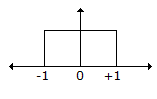33.

(29)13 ÷ (29)12 x (29)x = (23)16 Find x

 A. 11 B. 12 C. 13 D. 16

Explanation:

(29)13 ÷ (29)12 x (29)x = (23)16

=> (35)10 , (33)10 x (29)x = (35)10

=>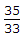x(29)X = 35

(29)x = (33)10 => X = 12.

34.

If the scattering matrix [S] of a two port network is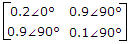then the network is

 A. lossless and reciprocal B. lossless but not reciprocal C. not lossless but reciprocal D. neither lossless nor reciprocal

Explanation:

S11 ≠ S22 ≠ lossy [S]1 = [S] Reciprocal.

35.

Consider the frequency modulated signal 10 cos (2p x 105t + 5 sin (2p x 1500t) + 7.5 sin (2p x 1000t) with carrier frequency of 105Hz. The modulation index is

 A. 12.5 B. 10 C. 7.5 D. 5

Explanation:

Total frequency deviation = 5 x 1500 + 7.5 x 1000 = 15000

Thus modulation index =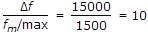.

#### Current Affairs 2022

Interview Questions and Answers